Next: 6.2.5 Training Up: 6.2 Methods Previous: 6.2.3 Image processing

## 6.2.4 Tuning curves

A training pattern combines visual and postural information. The visual part contains the activation of the 16 Gaussian position neurons and the edge-orientation histogram. Position and orientation were thus represented with a population code.

To obtain also a population code for each joint angle, an angle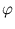was represented by the activation of four neurons with Gaussian receptive fields, ai = exp(- (-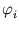)2/(2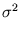)) (using a population code enhanced the performance, see section 6.3). Each of these Gaussians is a tuning curve tuned to the angle. The Gaussian centerswere uniformly distributed within the maximal range of each angle. The width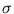was set equal to the distance between two neighboring centers (figure 6.6).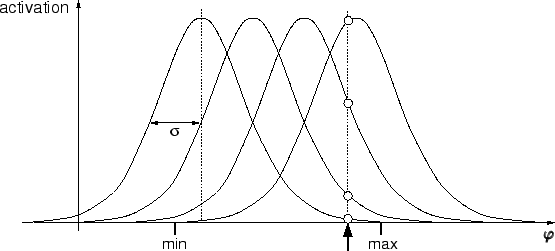All joint angles of the pre-grasping and the grasping posture were therefore encoded in 48 variables, which form the postural part of a training pattern. The final patterns were thus 68-dimensional. Before training, the patterns were normalized to have unit variance in each dimension. The resulting normalization constants were also applied to the test patterns.Next: 6.2.5 Training Up: 6.2 Methods Previous: 6.2.3 Image processing
Heiko Hoffmann
2005-03-22